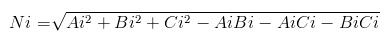# Thread: How to calculate the unbalanced neutral current in a 3 phase circuit

1.Junior Member
Join Date
Oct 2010
Posts
4
Mentioned
0 Post(s)
Tagged

## How to calculate the unbalanced neutral current in a 3 phase circuit

I was teaching a class in sharing the grounded conductor in a single phase circuit.
We only have 3 phase power in our class. I built a simple circuit with 2 equal loads on 2 seperate phases with a shared grounded conductor. I figured that the current in the grounded conductor in a three phase circuit would not be as low as in a single phase situation, but it would be significantly less than in the 2 hots.
When we measured the currents, the neutral was almost the same as the hots. what gives?
So does anyone know the formula for calculating the current in the grounded conductor in an unbalanced 3 phase circuit? I went over lots forums and archived forums and couldn't find any thing. I appreciate any help you can give.2.Senior Member
Join Date
Dec 2010
Location
-
Posts
1,508
Mentioned
0 Post(s)
Tagged3.4. Exactly the reason 310.15(B)(5)(b) exists.

(b)In a 3-wire circuit consisting of two phase conductors and the neutral conductor of a 4-wire, 3-phase, wye-connected system, a common conductor carries approximately the same current as the line-to-neutral load currents of the other conductors and shall be counted when applying the provisions of 310.15(B)(3)(a)5. Here is where vector mathematics comes in handy, and something you should be teaching to your students. Assuming resistive loads and all currents being equal in magnitude, you will have Ia = I∠120° and Ib = I∠240°. The sum of these two currents in the neutral will be found by adding the vectors. In this case, I chose to use the parallelogram method because it shows better in a single diagram. The other method is to redraw the vectors head-to-tail. (Don't ever let someone here tell you that tail-to-tail vectors means they should be subtracted. That is inappropriate vector math.)

Ia + Ib = I∠120° + I∠240° = I∠180°

In your example, you are missing the Ic = I∠0°. If Ic was present, then the total neutral current would be I∠180° + I∠0° = 06.Originally Posted by rmannWhen we measured the currents, the neutral was almost the same as the hots. what gives?
That question has been answered. But let me suggest that you take the same two phase wires that you used for your experiment and connect them instead to the primary of a single phase transformer. The voltage ratio is not important, but for discussion sake let us say the secondary voltage is 120/240. Try your experiment again, this time using the transformer secondary as your voltage source.7.Originally Posted by 480sparkyThat is fine where load power factor is identical on all three phases. But not if the power factors differ.8.Originally Posted by BesoekerThat is fine where load power factor is identical on all three phases. But not if the power factors differ.That is certainly a fact, but in any electricians training and testing I have seen they are looking for what 480 posted.

They don't usally consider PF in the questions involving neutral current.9.Originally Posted by iwireThat is certainly a fact, but in any electricians training and testing I have seen they are looking for what 480 posted.
They don't usally consider PF in the questions involving neutral current.
I'm sure what you say is correct. But it's a bit of a shame, I think. By all means give the expression that 480 posted but with the caveat that it applies to very specific circumstances, not all. Even not most.
Nah, on reflection, I don't think it should be taught to electricians. Is it of any practical use?
For the simple example I gave, the neutral current is nearly three times the magnitude that you would get by evaluating that expression.10.Originally Posted by BesoekerI'm sure what you say is correct. But it's a bit of a shame, I think. By all means give the expression that 480 posted but with the caveat that it applies to very specific circumstances, not all. Even not most.
Nah, on reflection, I don't think it should be taught to electricians. Is it of any practical use?
For the simple example I gave, the neutral current is nearly three times the magnitude that you would get by evaluating that expression.
Do not confuse the situation with real life facts, we get flustered3 phase, 3 phase 208 120 4 wire, calculations, neutral current, unbalanced load